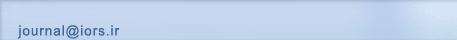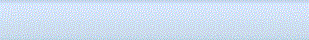[Home ] [Archive]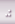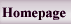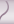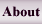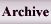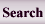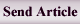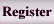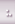Home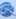Journal InformationArticles archiveSubmission InstructionRegistrationSubmit articleSite FacilitiesContact us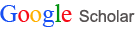All Since 2015 Citations 47945 18757 h-index 9 7 i10-index 9 4

Search in website
 Search the articlesSearch the site Advanced Search
 Enter your Email in the following box to receive the site news and information.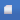Search published articles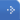Showing 2 results for Jain

Jain, Lachhwani,
Volume 2, Issue 1 (vol 2. No 1 2010)
Abstract

We develop an algorithm for the solution of multiobjective linear plus fractional programming problem (MOL+FPP) when some of the constraints are homogeneous in nature. Using homogeneous constraints, first we construct a transformation matrix T which transforms the given problem into another MOL+FPP with fewer constraints. Then, a relationship between these two problems, ensuring that the solution of the original problem can be recovered from the solution of the transformed problem, is established. We repeat this process of transformation until all the homogeneous constraints are removed. Then, we discuss the multi objective programming part, for which fuzzy programming methodology is proposed which works for the minimization of perpendicular distances between two hyper planes (curves) at the optimal points of the objective functions. A suitable membership function is defined with the help of the supremum perpendicular distance. A compromised optimal solution is obtained as a result of the minimization of the The supremum perpendicular distance. The corresponding optimal solution to the original problem is obtained using the transformation matrix. Finally, an example is given to illustrate the proposed model.

Jain,
Volume 2, Issue 1 (vol 2. No 1 2010)
Abstract

The fuzzy approach has undergone a profound structural transformation in the past few decades. Numerous studies have been undertaken to explain fuzzy approach for linear and nonlinear programs. While, the findings in earlier studies have been conflicting, recent studies of competitive situations indicate that fractional programming problem has a positive impact on comparative scenario. We propose one of the best interval approximations, close interval approximation of piecewise quadratic fuzzy numbers for solving fuzzy number fractional programming problem without converting it to a crisp problem. A new form of simplex method is introduced here for solving fuzzy number fractional programming problem using fuzzy arithmetic. The fuzzy analogue of some important theorems of fuzzy fractional programming problem proved. A fuzzy fractional programming problem is worked out as an example to illustrate the proposed method.

 Page 1 from 1